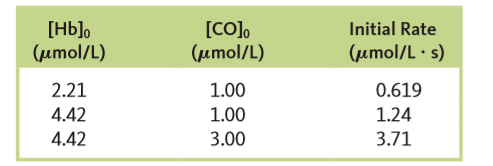# Problem: The rate of the reaction between hemoglobin (Hb) and carbon monoxide (CO) was studied at 20°C. The following data were collected with all concentration units in μmol/L. (A hemoglobin concentration of 2.21 μmol/L is equal to 2.21 x 10-6 mol/L.)a. Determine the orders of this reaction with respect to Hb and CO.

###### FREE Expert Solution

(1) For Hb, use experiment 1 and 2.

82% (16 ratings)###### Problem Details

The rate of the reaction between hemoglobin (Hb) and carbon monoxide (CO) was studied at 20°C. The following data were collected with all concentration units in μmol/L. (A hemoglobin concentration of 2.21 μmol/L is equal to 2.21 x 10-6 mol/L.)a. Determine the orders of this reaction with respect to Hb and CO.

Frequently Asked Questions

What scientific concept do you need to know in order to solve this problem?

Our tutors have indicated that to solve this problem you will need to apply the Rate Law concept. You can view video lessons to learn Rate Law. Or if you need more Rate Law practice, you can also practice Rate Law practice problems.

What professor is this problem relevant for?

Based on our data, we think this problem is relevant for Professor Detwiler's class at UW-SEATTLE.

What textbook is this problem found in?

Our data indicates that this problem or a close variation was asked in Chemistry: An Atoms First Approach - Zumdahl Atoms 1st 2nd Edition. You can also practice Chemistry: An Atoms First Approach - Zumdahl Atoms 1st 2nd Edition practice problems.